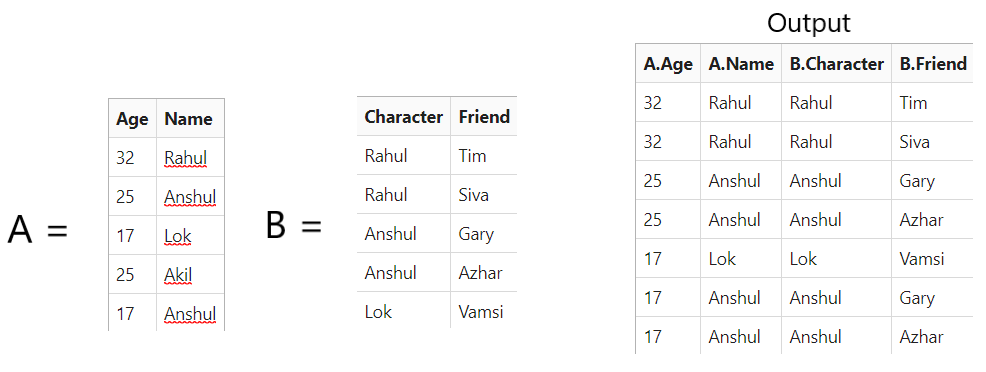# Joining Tables using MultiMaps

• Last Updated : 25 Oct, 2020

Joining two different tables on their matching columns can be done using nested loops, but a more efficient and scalable way is to use multimaps. The idea is to map from each column value that we want to join to all the rows that contain it, to generate a multimap from a table out of both tables.

The multimap generated has to be hash-based. Hashing is essentially a technique converting a large element into a small element that represents the same element. Therefore, generate the multimap for the smaller table, thus decreasing its generation time and memory size.

Attention reader! Don’t stop learning now. Get hold of all the important DSA concepts with the DSA Self Paced Course at a student-friendly price and become industry ready.  To complete your preparation from learning a language to DS Algo and many more,  please refer Complete Interview Preparation Course.

In case you wish to attend live classes with experts, please refer DSA Live Classes for Working Professionals and Competitive Programming Live for Students.

Example:Approach:

• Create the two tables.
• Now, get the ID of the columns on both of the tables.
• Then create and implement a multimap for mapping to various rows of table B.
• Print the result after the above steps.

Below is the implementation of the above approach:

## C++

 `// C++ program for hashjoin on two tables``#include ``#include ``#include ``#include ``using` `namespace` `std;`` ` `// Generate two tables to join``using` `tab_t = vector >;`` ` `// Table 1``tab_t tab1{ ``// Age  Name``            ``{ ``"32"``, ``"Rahul"` `},``            ``{ ``"25"``, ``"Anshul"` `},``            ``{ ``"17"``, ``"Lok"` `},``            ``{ ``"25"``, ``"Akil"` `},``            ``{ ``"17"``, ``"Anshul"` `}``};`` ` `// Table 2``tab_t tab2{ ``// Student  Friend``            ``{ ``"Rahul"``, ``"Tim"` `},``            ``{ ``"Rahul"``, ``"Siva"` `},``            ``{ ``"Anshul"``, ``"Gary"` `},``            ``{ ``"Anshul"``, ``"Azhar"` `},``            ``{ ``"Lok"``, ``"Vamsi"` `}``};`` ` `// Overloading of Output Operator``ostream& operator<<(ostream& o,``                    ``const` `tab_t& t)``{``    ``// Iterate through the tablt t``    ``for` `(``size_t` `i = 0; i < t.size(); ++i) {``        ``o << i << ``":"``;``        ``for` `(``const` `auto``& e : t[i])``            ``o << ``'\t'` `<< e;``        ``o << endl;``    ``}``    ``return` `o;``}`` ` `// Function that perform join operation``// on the two tables``tab_t Join(``const` `tab_t& a, ``size_t` `columna,``           ``const` `tab_t& b, ``size_t` `columnb)``{``    ``unordered_multimap hashmap;`` ` `    ``// Use of Hashmap``    ``for` `(``size_t` `i = 0;``         ``i < a.size(); ++i) {``        ``hashmap.insert({ a[i][columna], i });``    ``}`` ` `    ``// Perform Mapping``    ``tab_t result;``    ``for` `(``size_t` `i = 0; i < b.size(); ++i) {`` ` `        ``auto` `range = hashmap.equal_range(``            ``b[i][columnb]);`` ` `        ``// Create new joined table``        ``for` `(``auto` `it = range.first;``             ``it != range.second; ++it) {`` ` `            ``tab_t::value_type row;`` ` `            ``// Insert values to row``            ``row.insert(row.end(),``                       ``a[it->second].begin(),``                       ``a[it->second].end());``            ``row.insert(row.end(),``                       ``b[i].begin(),``                       ``b[i].end());`` ` `            ``// Push the row``            ``result.push_back(move(row));``        ``}``    ``}``    ``return` `result;``}`` ` `// Driver Code``int` `main(``int` `argc, ``char` `const``* argv[])``{``    ``int` `ret = 0;`` ` `    ``// Given Tables``    ``cout << ``"Table A: "` `<< endl``         ``<< tab1 << endl;``    ``cout << ``"Table B: "` `<< endl``         ``<< tab2 << endl;`` ` `    ``// Function Call``    ``auto` `tab3 = Join(tab1, 1, tab2, 0);`` ` `    ``// Print the joined table``    ``cout << ``"Joined tables: "` `<< endl``         ``<< tab3 << endl;``    ``return` `ret;``}`

## Python3

 `# Python program for hashjoin on two tables``from` `collections ``import` `defaultdict`` ` `# Function that perform join operation``# on the two tables``def` `hashJoin(table1, index1, table2, index2):`` ` `    ``h ``=` `defaultdict(``list``)`` ` `    ``# Hash``    ``for` `s ``in` `table1:``        ``h[s[index1]].append(s)`` ` `    ``# Perform join operation``    ``return` `[(s, r) ``for` `r ``in` `table2 ``for` `s ``in` `h[r[index2]]]`` ` `# Driver Code`` ` `# Given two tables``table1 ``=` `[(``"32"``, ``"Rahul"``),``           ``(``"25"``, ``"Anshul"``),``           ``(``"17"``, ``"Lok"``),``           ``(``"25"``, ``"Akil"``),``           ``(``"17"``, ``"Anshul"``)]`` ` `table2 ``=` `[(``"Rahul"``, ``"Tim"``),``          ``(``"Rahul"``, ``"Siva"``),``          ``(``"Anshul"``, ``"Gary"``),``          ``(``"Anshul"``, ``"Azhar"``),``          ``(``"Lok"``, ``"Vamsi"``)]`` ` `# Print the resultant table``for` `row ``in` `hashJoin(table1, ``1``, table2, ``0``):``    ``print``(row)`
Output:
```Table A:
0:    32    Rahul
1:    25    Anshul
2:    17    Lok
3:    25    Akil
4:    17    Anshul

Table B:
0:    Rahul    Tim
1:    Rahul    Siva
2:    Anshul    Gary
3:    Anshul    Azhar
4:    Lok    Vamsi

Joined tables:
0:    32    Rahul    Rahul    Tim
1:    32    Rahul    Rahul    Siva
2:    17    Anshul    Anshul    Gary
3:    25    Anshul    Anshul    Gary
4:    17    Anshul    Anshul    Azhar
5:    25    Anshul    Anshul    Azhar
6:    17    Lok    Lok    Vamsi
```

My Personal Notes arrow_drop_up